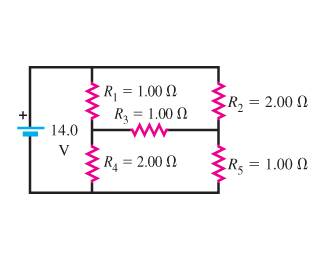# Finding current in a complex circuit with resistors

I am given the following circuit:How do I find the current through the battery? I have trouble seeing which resistors are in series and which resistors are in parallel. I think I need to figure that out and then calculate R_eq accordingly. Then set I=V/R and solve for I.

But which resistors are in series and which are in parallel? It's confusing the way the circuit is laid out here...

Galileo
Homework Helper
Use conservation of charge (KCL) at the two nodes across R3.

nrqed
Homework Helper
Gold Member
Goomba said:
I am given the following circuit:How do I find the current through the battery? I have trouble seeing which resistors are in series and which resistors are in parallel. I think I need to figure that out and then calculate R_eq accordingly. Then set I=V/R and solve for I.

But which resistors are in series and which are in parallel? It's confusing the way the circuit is laid out here...

No two resistors are in series nor in parallel here. There is no way to simplify. You must solve the entire circuit using Kirchhoff's rules. Find the current flowing out of the battery (no need to find the other currents). Then just use R_eq = V_battery / I_ out of the battery.

Pat

Thanks

I figured it out.The node-voltage method is a beautiful thing.

u can also use the star-delta transformation to simplify the circuit given